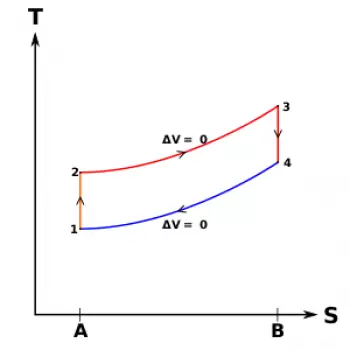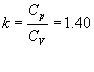# Theoretical Cycles of Heat EnginesTheoretical cycles are approximations to the real cycles. They are used to study the performance of an engine. In a real cycle there are so many variables that affect the efficiency of the engine that it would be impossible to make exact calculations. However, theoretical cycles allow us to make very reliable approximations.

For endothermic motor cycles, the most used approximations in order of approximation to the real conditions are three:

• Actual cycle or indicated cycle, measured empirically.
• Air cycle
• Air-fuel cycle.

These theoretical cycles are compared in practice with real cycles. The real cycles are obtained experimentally by means of the indicators. The use of these indicators is the reason why real cycles are also called indicated cycles.

Theoretical cycles are not identical to real cycles, but they are a very useful tool for studying internal combustion engines thermodynamically. Especially to understand how much the operating conditions influence its use and to compare different types of motors with each other.

## Assumptions of Theoretical Cycles

Theoretical cycles can also be called ideal cycles since it is calculated assuming that the operating conditions are ideal as detailed below.

In theoretical cycles (or ideal cycles) it is assumed that the operating fluid is constituted by air and that it behaves like a perfect gas. For this reason, the values ​​of the specific heats are considered constant and equal to those of the air at conditions such as 15º of temperature and 1 atmosphere of pressure:

Cp = 0.241 Ca / kg ° C;
Cv = 0.172 Ca / kg ° C;

Where it results:We also assume that in the theoretical cycle (or ideal cycle) the heat introduction and subtraction phases have a well-determined duration, depending on the type of cycle ( Otto cycle, diesel cycle, Sabbath cycle), and that in the others phases there are no heat losses.

With these assumptions, the maximum values ​​of temperature and pressure, as well as the work and thermal efficiency calculated for the theoretical cycle (or ideal cycle), are higher than those corresponding to the other types of cycles.

The theoretical cycle (or ideal cycle) represents the maximum limit that the engine can theoretically reach and allows an easy mathematical study based on the perfect gas laws.

## What Is the Air Cycle of a Heat Engine?

In the air cycle, the operating fluid is also air, but the specific heats are assumed to be variable throughout the temperature range in which it operates.

The conditions for introducing and removing heat are the same as in the ideal cycle and there are no heat losses. Since the calculation of the thermodynamic variables of the mean specific heats is complicated, tables are used that directly give the values ​​of heat and work. These energy values ​​are in terms of internal energy and enthalpy for the various points of the isentropic transformations of the air. Taking into account the variations in specific heats, values ​​lower than those calculated for the ideal cycle are obtained for maximum temperatures and pressures; consequently, the work and the thermal efficiency also lower. However, they are still larger than those corresponding to a real cycle.

## Air-fuel Cycle in a Heat Engine

Among all the cycles that are calculated, the air-fuel cycle is the closest to the real cycle. In the positive ignition engine (Otto cycle), the fluid is composed, during the suction phase, of the mixture and the residual gases of the previous combustion. In the compression ignition engine it is made up of air and waste gases. After combustion, the fluid is made up of its products, that is, a mixture of CO 2 , CO, H 2 O, N 2 .

These gases have an average specific heat even higher than that of air; but in addition, there is a subsequent increase in specific heats. This increase is due to the dissociation or chemical decomposition of the lighter molecules subjected to the action of high temperatures. The increase in specific heats, as well as the dissociation that, being an endothermic reaction, absorbs a part of the heat of combustion, produce a subsequent decrease in temperature and maximum pressure compared to those calculated for the air cycle.

Tables containing data obtained experimentally are used to calculate the air-fuel cycle. Even for this cycle it is admitted not only that heat is introduced and subtracted instantaneously, as in the ideal cycle, but that no heat losses occur.

## What Is the Real Cycle of Endothermic Engines?

The real cycle is obtained experimentally. The actual cycle is carried out by means of various indicating devices, capable of recording the diagram of pressures as a function of volumes, in a working power cylinder.

The diagram indicated reflects the real conditions of the cycle and, therefore, also takes into account - in addition to the variations already stated for the air cycle and for the air-fuel cycle in the comparison of ideal cycles - the heat losses, the duration combustion, the losses caused by fluid friction, the duration of the valve opening time, the ignition time, as well as the injection time and the exhaust losses.

Author:

Published: December 10, 2009
Last review: February 16, 2020